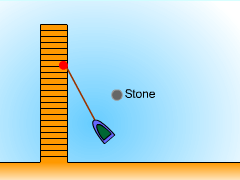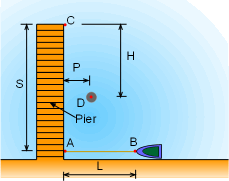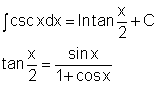Ch 8. Integration Techniques Multimedia Engineering Math Integration byParts Trig. IntegralsTrig. Subst. Fractions& Substitutions ApproximateIntegration
 Chapter 1. Limits 2. Derivatives I 3. Derivatives II 4. Mean Value 5. Curve Sketching 6. Integrals 7. Inverse Functions 8. Integration Tech. 9. Integrate App. 10. Parametric Eqs. 11. Polar Coord. 12. Series Appendix Basic Math Units Search eBooks Dynamics Fluids Math Mechanics Statics Thermodynamics Author(s): Hengzhong Wen Chean Chin Ngo Meirong Huang Kurt Gramoll ©Kurt GramollMATHEMATICS - CASE STUDY IntroductionMotion of the RowboatLocation of the Stone In a park, a boat is initially stayed beside the pier by a rope of length L at point A (the rope is loose). The the wind blows the boat to point B (the rope is straight and tout). The worker needs to pull the boat to the end of the pier (point C) to load people. A big stone is located at point D. The worker walks along the pier from point A toward point C and pulls the rowboat by the rope with him. During the pulling, the man keeps the rope straight and tout.  What is known: The length of the rope is L = 20 m.   The distance from A to C is S = 50 m. The stone located at point D which is  5 m from the pier and 25 m from the bridge. Neglect the size of the stone and the rowboat.  Rope is not pulled in until the man reaches the end of the pier. Questions Determine if the boat will collide with the stone. Approach Since the man pulls the boat by a rope which is straight and tout, the path followed by the boat will be a curve and the rope will always be tangent to the curve. After the man arrives the bridge, he stands there to pull the boat to the bridge. Consider trigonometric substitutions